JMSLTM Numerical Library 7.2.0
com.imsl.stat

## Class StepwiseRegression

• All Implemented Interfaces:
Serializable, Cloneable

```public class StepwiseRegression
extends Object
implements Serializable, Cloneable```
Builds multiple linear regression models using forward selection, backward selection, or stepwise selection.

Class `StepwiseRegression` builds a multiple linear regression model using forward selection, backward selection, or forward stepwise (with a backward glance) selection.

Levels of priority can be assigned to the candidate independent variables using the `setLevels(int[])` method. All variables with a priority level of 1 must enter the model before variables with a priority level of 2. Similarly, variables with a level of 2 must enter before variables with a level of 3, etc. Variables also can be forced into the model (`setForce(int)`). Note that specifying "force" without also specifying the levels will result in all variables being forced into the model.

Typically, the intercept is forced into all models and is not a candidate variable. In this case, a sum-of-squares and crossproducts matrix for the independent and dependent variables corrected for the mean is required. Other possibilities are as follows:

1. The intercept is not in the model. A raw (uncorrected) sum-of-squares and crossproducts matrix for the independent and dependent variables is required as input in `cov`. Argument `nObservations` must be set to one greater than the number of observations.
2. An intercept is a candidate variable. A raw (uncorrected) sum-of-squares and crossproducts matrix for the constant regressor (=1), independent and dependent variables are required for `cov`. In this case, `cov` contains one additional row and column corresponding to the constant regressor. This row/column contains the sum-of-squares and crossproducts of the constant regressor with the independent and dependent variables. The remaining elements in `cov` are the same as in the previous case. Argument `nObservations` must be set to one greater than the number of observations.

The stepwise regression algorithm is due to Efroymson (1960). `StepwiseRegression` uses sweeps of the covariance matrix (input in `cov`, if the covariance matrix is specified, or generated internally) to move variables in and out of the model (Hemmerle 1967, Chapter 3). The SWEEP operator discussed in Goodnight (1979) is used. A description of the stepwise algorithm is also given by Kennedy and Gentle (1980, pp. 335-340). The advantage of stepwise model building over all possible regression (`SelectionRegression`) is that it is less demanding computationally when the number of candidate independent variables is very large. However, there is no guarantee that the model selected will be the best model (highest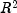) for any subset size of independent variables.

Example 1, Serialized Form
• ### Nested Class Summary

Nested Classes
Modifier and Type Class and Description
`class ` `StepwiseRegression.CoefficientTTests`
`CoefficientTTests` contains statistics related to the student-t test, for each regression coefficient.
`static class ` `StepwiseRegression.CyclingIsOccurringException`
Cycling is occurring.
`static class ` `StepwiseRegression.NoVariablesEnteredException`
No Variables can enter the model.
• ### Field Summary

Fields
Modifier and Type Field and Description
`static int` `BACKWARD_REGRESSION`
Indicates backward regression.
`static int` `FORWARD_REGRESSION`
Indicates forward regression.
`static int` `STEPWISE_REGRESSION`
Indicates stepwise regression.
• ### Constructor Summary

Constructors
Constructor and Description
```StepwiseRegression(double[][] x, double[] y)```
Creates a new instance of `StepwiseRegression`.
```StepwiseRegression(double[][] x, double[] y, double[] weights)```
Creates a new instance of weighted `StepwiseRegression`.
```StepwiseRegression(double[][] x, double[] y, double[] weights, double[] frequencies)```
Creates a new instance of weighted `StepwiseRegression` using observation frequencies.
```StepwiseRegression(double[][] cov, int nObservations)```
Creates a new instance of `StepwiseRegression` from a user-supplied variance-covariance matrix.
• ### Method Summary

Methods
Modifier and Type Method and Description
`void` `compute()`
Builds the multiple linear regression models using forward selection, backward selection, or stepwise selection.
`ANOVA` `getANOVA()`
Get an analysis of variance table and related statistics.
`StepwiseRegression.CoefficientTTests` `getCoefficientTTests()`
Returns the student-t test statistics for the regression coefficients.
`double[]` `getCoefficientVIF()`
Returns the variance inflation factors for the final model in this invocation.
`double[][]` `getCovariancesSwept()`
Returns the results after `cov` has been swept for the columns corresponding to the variables in the model.
`double[]` `getHistory()`
Returns the stepwise regression history for the independent variables.
`double` `getIntercept()`
Returns the intercept.
`double[]` `getSwept()`
Returns an array containing information indicating whether or not a particular variable is in the model.
`void` `setForce(int force)`
Forces independent variables into the model based on their level assigned from `setlevels`.
`void` `setLevels(int[] levels)`
Sets the levels of priority for variables entering and leaving the regression.
`void` `setMeans(double[] means)`
Sets the means of the variables.
`void` `setMethod(int method)`
Specifies the stepwise selection method, forward, backward, or stepwise Regression.
`void` `setPValueIn(double pValueIn)`
Defines the largest p-value for variables entering the model.
`void` `setPValueOut(double pValueOut)`
Defines the smallest p-value for removing variables.
`void` `setTolerance(double tolerance)`
The tolerance used to detect linear dependence among the independent variables.
• ### Methods inherited from class java.lang.Object

`clone, equals, finalize, getClass, hashCode, notify, notifyAll, toString, wait, wait, wait`
• ### Field Detail

• #### BACKWARD_REGRESSION

`public static final int BACKWARD_REGRESSION`
Indicates backward regression. An attempt is made to remove a variable from the model. A variable is removed if its p-value is greater than `pValueOut`. During initialization, all candidate independent variables enter the model.
Constant Field Values
• #### FORWARD_REGRESSION

`public static final int FORWARD_REGRESSION`
Indicates forward regression. An attempt is made to add a variable to the model. A variable is added if its p-value is less than `pValueIn`. During intitialization, only forced variables enter the model.
Constant Field Values
• #### STEPWISE_REGRESSION

`public static final int STEPWISE_REGRESSION`
Indicates stepwise regression. A backward step is attempted. After the backward step, a forward step is attempted. This is a stepwise step. Any forced variables enter the model during initialization.
Constant Field Values
• ### Constructor Detail

• #### StepwiseRegression

```public StepwiseRegression(double[][] x,
double[] y)
throws com.imsl.stat.Covariances.TooManyObsDeletedException,
com.imsl.stat.Covariances.MoreObsDelThanEnteredException,
com.imsl.stat.Covariances.DiffObsDeletedException```
Creates a new instance of `StepwiseRegression`.
Parameters:
`x` - a `double` matrix of nObs by nVars, where nObs is the number of observations and nVars is the number of independent variables.
`y` - a `double` array containing the observations of the dependent variable.
Throws:
`com.imsl.stat.Covariances.TooManyObsDeletedException` - is thrown if more observations have been deleted than were originally entered, i.e. the sum of frequencies has become negative.
`com.imsl.stat.Covariances.MoreObsDelThanEnteredException` - is thrown if more observations are being deleted from "variance-covariance" matrix than were originally entered. The corresponding row, column of the incidence matrix is less than zero.
`com.imsl.stat.Covariances.DiffObsDeletedException` - is thrown if different observations are being deleted than were originally entered.
• #### StepwiseRegression

```public StepwiseRegression(double[][] x,
double[] y,
double[] weights)
throws Covariances.NonnegativeWeightException,
com.imsl.stat.Covariances.TooManyObsDeletedException,
com.imsl.stat.Covariances.MoreObsDelThanEnteredException,
com.imsl.stat.Covariances.DiffObsDeletedException```
Creates a new instance of weighted `StepwiseRegression`.
Parameters:
`x` - a `double` matrix of nObs by nVars, where nObs is the number of observations and nVars is the number of independent variables.
`y` - a `double` array containing the observations of the dependent variable.
`weights` - a `double` array containing the weight for each observation of `x`.
Throws:
`Covariances.NonnegativeWeightException` - is thrown if the weights are negative.
`com.imsl.stat.Covariances.TooManyObsDeletedException` - is thrown if more observations have been deleted than were originally entered, i.e. the sum of frequencies has become negative.
`com.imsl.stat.Covariances.MoreObsDelThanEnteredException` - is thrown if more observations are being deleted from "variance-covariance" matrix than were originally entered. The corresponding row, column of the incidence matrix is less than zero.
`com.imsl.stat.Covariances.DiffObsDeletedException` - is thrown if different observations are being deleted than were originally entered.
• #### StepwiseRegression

```public StepwiseRegression(double[][] x,
double[] y,
double[] weights,
double[] frequencies)
throws Covariances.NonnegativeFreqException,
Covariances.NonnegativeWeightException,
com.imsl.stat.Covariances.TooManyObsDeletedException,
com.imsl.stat.Covariances.MoreObsDelThanEnteredException,
com.imsl.stat.Covariances.DiffObsDeletedException```
Creates a new instance of weighted `StepwiseRegression` using observation frequencies.
Parameters:
`x` - a `double` matrix of nObs by nVars, where nObs is the number of observations and nVars is the number of independent variables.
`y` - a `double` array containing the observations of the dependent variable.
`weights` - a `double` array containing the weight for each observation of `x`.
`frequencies` - a `double` array containing the frequency for each row of `x`.
Throws:
`Covariances.NonnegativeFreqException` - is thrown if the frequencies are negative.
`Covariances.NonnegativeWeightException` - is thrown if the weights are negative.
`com.imsl.stat.Covariances.TooManyObsDeletedException` - is thrown if more observations have been deleted than were originally entered, i.e. the sum of frequencies has become negative.
`com.imsl.stat.Covariances.MoreObsDelThanEnteredException` - is thrown if more observations are being deleted from "variance-covariance" matrix than were originally entered. The corresponding row, column of the incidence matrix is less than zero.
`com.imsl.stat.Covariances.DiffObsDeletedException` - is thrown if different observations are being deleted than were originally entered.
• #### StepwiseRegression

```public StepwiseRegression(double[][] cov,
int nObservations)```
Creates a new instance of `StepwiseRegression` from a user-supplied variance-covariance matrix.
Parameters:
`cov` - a `double` matrix containing a variance-covariance or sum of squares and crossproducts matrix, in which the last column must correspond to the dependent variable. `cov` can be computed using the `Covariances` class.
`nObservations` - an `int` containing the number of observations associated with `cov`.
• ### Method Detail

• #### compute

```public void compute()
throws StepwiseRegression.NoVariablesEnteredException,
StepwiseRegression.CyclingIsOccurringException```
Builds the multiple linear regression models using forward selection, backward selection, or stepwise selection.
Throws:
`StepwiseRegression.NoVariablesEnteredException` - is thrown if no variables entered the model. All elements of `ANOVA` table are set to `NaN`
`StepwiseRegression.CyclingIsOccurringException` - is thrown if cycling occurs
• #### getANOVA

```public ANOVA getANOVA()
throws StepwiseRegression.NoVariablesEnteredException,
StepwiseRegression.CyclingIsOccurringException```
Get an analysis of variance table and related statistics.
Returns:
an `ANOVA` table and related statistics.
Throws:
`StepwiseRegression.NoVariablesEnteredException`
`StepwiseRegression.CyclingIsOccurringException`
• #### getCoefficientTTests

```public StepwiseRegression.CoefficientTTests getCoefficientTTests()
throws StepwiseRegression.NoVariablesEnteredException,
StepwiseRegression.CyclingIsOccurringException```
Returns the student-t test statistics for the regression coefficients.
Returns:
a `StepwiseRegression.CoefficientTTests` object containing statistics relating to the regression coefficients.
Throws:
`StepwiseRegression.NoVariablesEnteredException`
`StepwiseRegression.CyclingIsOccurringException`
• #### getCoefficientVIF

```public double[] getCoefficientVIF()
throws StepwiseRegression.NoVariablesEnteredException,
StepwiseRegression.CyclingIsOccurringException```
Returns the variance inflation factors for the final model in this invocation. The elements are in the same order as the independent variables in `x` (or, if the covariance matrix is specified, the elements are in the same order as the variables in ```cov ```). Each element corresponding to a variable not in the model contains statistics for a model which includes the variables of the final model and the variables corresponding to the element in question.

The square of the multiple correlation coefficient for the i-th regressor after all others can be obtained from the i-th element for the returned array by the following formula: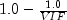Returns:
a `double` array containing the variance inflation factors for the final model in this invocation.
Throws:
`StepwiseRegression.NoVariablesEnteredException`
`StepwiseRegression.CyclingIsOccurringException`
• #### getCovariancesSwept

```public double[][] getCovariancesSwept()
throws StepwiseRegression.NoVariablesEnteredException,
StepwiseRegression.CyclingIsOccurringException```
Returns the results after `cov` has been swept for the columns corresponding to the variables in the model.
Returns:
a `double` matrix containing the results after `cov` has been swept on the columns corresponding to the variables in the model. The estimated variance-covariance matrix of the estimated regression coefficients in the final model can be obtained by extracting the rows and columns corresponding to the independent variables in the final model and multiplying the elements of this matrix by the error mean square.
Throws:
`StepwiseRegression.NoVariablesEnteredException`
`StepwiseRegression.CyclingIsOccurringException`
• #### getHistory

```public double[] getHistory()
throws StepwiseRegression.NoVariablesEnteredException,
StepwiseRegression.CyclingIsOccurringException```
Returns the stepwise regression history for the independent variables.
Returns:
a `double` array containing the recent history of the independent variables. The last element corresponds to the dependent variable.

 history[i] Status of i-th Variable 0.0 This variable has never been added to the model. 0.5 This variable was added into the model during initialization. k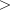0.0 This variable was added to the model during the k-th step. k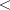0.0 This variable was deleted from model during the k-th step

Throws:
`StepwiseRegression.NoVariablesEnteredException`
`StepwiseRegression.CyclingIsOccurringException`
`setLevels(int[])`
• #### getIntercept

```public double getIntercept()
throws StepwiseRegression.NoVariablesEnteredException,
StepwiseRegression.CyclingIsOccurringException```
Returns the intercept. The intercept is computed as follows: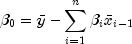where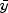is the mean of the dependent variable `y`,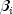are the coefficients, and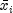are the mean values for each independent variable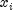in the final model. If the covariance matrix is used for input, use method `setMean` to specify the means of the variables. If `x` and `y` are used for input, the means are computed internally and do not need to be specified.
Returns:
a `double` containing the intercept.
Throws:
`StepwiseRegression.NoVariablesEnteredException`
`StepwiseRegression.CyclingIsOccurringException`
• #### getSwept

```public double[] getSwept()
throws StepwiseRegression.NoVariablesEnteredException,
StepwiseRegression.CyclingIsOccurringException```
Returns an array containing information indicating whether or not a particular variable is in the model.
Returns:
a `double` array with information to indicate the independent variables in the model. The last element corresponds to the dependent variable. A +1 in the i-th position indicates that the variable is in the selected model. A -1 indicates that the variable is not in the selected model.
Throws:
`StepwiseRegression.NoVariablesEnteredException`
`StepwiseRegression.CyclingIsOccurringException`
`setLevels(int[])`
• #### setForce

`public void setForce(int force)`
Forces independent variables into the model based on their level assigned from `setlevels`.
Parameters:
`force` - an `int` specifying the upper bound on the variables forced into the model. Variables with levels 1, 2, ..., `force` are forced into the model as independent variables.
`setLevels(int[])`
• #### setLevels

`public void setLevels(int[] levels)`
Sets the levels of priority for variables entering and leaving the regression. Each variable is assigned a positive value which indicates its level of entry into the model. A variable can enter the model only after all variables with smaller nonzero levels of entry have entered. Similarly, a variable can only leave the model after all variables with higher levels of entry have left. Variables with the same level of entry compete for entry (deletion) at each step. Argument `levels[i]=0` means the i-th variable never enters the model. Argument `levels[i]=-1` means the i-th variable is the dependent variable. The last element in `levels` must correspond to the dependent variable, except when the variance-covariance or sum of squares and crossproducts matrix is supplied.
Parameters:
`levels` - an `int` array containing the levels of entry into the model for each variable. Default: 1, 1, ..., 1, -1 where -1 corresponds to the dependent variable.
`setForce(int)`
• #### setMeans

`public void setMeans(double[] means)`
Sets the means of the variables. This is required when the covariance array is input and the intercept `getIntercept()` is requested. Otherwise, it is not used.
Parameters:
`means` - a `double` array of length nVars+1, where nVars is the number of independent variables. `means` through ``` means[nVars-1]``` are the means of the independent variables and ```means[nVars] ``` is the mean of the dependent variable.
`getIntercept()`
• #### setMethod

`public void setMethod(int method)`
Specifies the stepwise selection method, forward, backward, or stepwise Regression.
Parameters:
`method` - an `int` value between -1 and 1 specifying the stepwise selection method. Fields ``` FORWARD_REGRESSION```, ```BACKWARD_REGRESSION ```, and `STEPWISE_REGRESSION` should be used. Default: `STEPWISE_REGRESSION`.
`FORWARD_REGRESSION`, `BACKWARD_REGRESSION`, `STEPWISE_REGRESSION`
• #### setPValueIn

`public void setPValueIn(double pValueIn)`
Defines the largest p-value for variables entering the model. Variables with p-value less than `pValueIn` may enter the model. Backward regression does not use this value.
Parameters:
`pValueIn` - a `double` containing the largest p-value for variables entering the model. Default: `pValueIn` = 0.05.
• #### setPValueOut

`public void setPValueOut(double pValueOut)`
Defines the smallest p-value for removing variables. Variables with p-values greater than `pValueOut` may leave the model. `pValueOut` must be greater than or equal to `pValueIn`. A common choice for `pValueOut` is 2*`pValueIn`. Forward regression does not use this value.
Parameters:
`pValueOut` - a `double` containing the smallest p-value for removing variables from the model. Default: `pValueOut` = 0.10.
• #### setTolerance

`public void setTolerance(double tolerance)`
The tolerance used to detect linear dependence among the independent variables.
Parameters:
`tolerance` - a `double` containing the tolerance used for detecting linear dependence. Default: `tolerance` = 2.2204460492503e-16.
JMSLTM Numerical Library 7.2.0